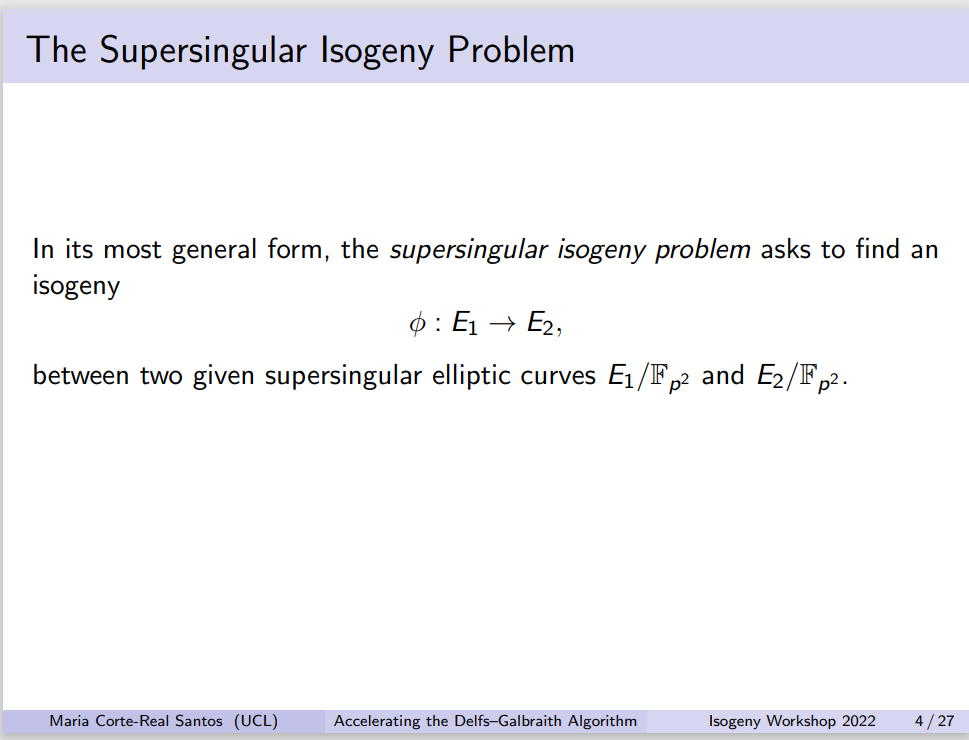【后量子密码】超奇异椭圆曲线同源密钥协商理论研究

SIDH:Supersingular Isogeny Diffie-Hellman

# 1.超奇异椭圆曲线

## 1.1.同构

isomorphism## 1.2.扭子群

torsion group

$E[m] = ker([m]) = \{ p \in E(\overline{K}) | mP = O\}$

E的扭子群

$E_{tors} = \bigcup_{m=1}^{\infty} E[m]$

$p \nmid m$ 时，$E[m] \cong (Z/mZ)^2$ ，对于 r ≥ 1 ，$E[p^r]$有两种情况：

(1) $E[p^r] = 0$ 对任意 r 同时成立

(2) $E[p^r] = Z/p^rZ$ 对任意 r 同时成立

## 1.3.超奇异

supersingular

$q = p^r$ , p为素数，r为正整数

# 2.同源

## 2.0.定义

isogeny

isogenous

$\phi:E_1 \rightarrow E_2$ 是椭圆曲线间的态射(morphism)且 $\phi(O_{E_1}) = O_{E_2}$，则称 $\phi$ 是椭圆曲线间的同源(isogeny)

(1) $\phi(E_1) = O$ , 此时称为零同源

(2) $\phi(E_1) = E_2$，此时 $K(E_1)$$K(E_2)$ 的有限扩张，且 $\phi$ 是有限加法满同态，故 $ker(\phi)$ 是有限子群

SIDH仅考虑后者

isogenous是等价关系。

Tate定理：

$E_1$, $E_2$ 为有限域 $F_q$ 上的椭圆曲线，则存在定义在 $F_q$ 上的同源 $\phi:E_1 \rightarrow E_2$ 当且仅当 $\#E_1(F_q)=\#E_2(F_q)$

$\Phi$$E$ 的一个有限子群，有且仅有一条椭圆曲线 E’ 和一个可分同源(separable isogeny)

$\phi:E \rightarrow E'$ 满足 $ker(\phi) = \Phi$

$\phi:E_1 \rightarrow E_2$ 是一个非零同源，那么

$ker \phi = \phi^{-1}(O)$ 是一个有限群

## 2.1.自同态环(*)

$E_1$$E_2$ 的所有isogeny的集合定义为

$Hom(E_1,E_2) = \{all\ isogenies :E_1 \rightarrow E_2\}$

$(\phi+\psi)(P) = \phi(P) + \psi(P)$

$End(E) = Hom(E,E)$

$(\phi\psi)(P) = \phi(\psi(P))$

## 2.2.乘以 m 同源

$[m]:E \rightarrow E$

[m] 的 kernel 即为 E[m] (E的m阶扭子群)

$\#E[m] = m^2 , E[m] \cong (Z/dZ) \times (Z/mZ)$

## 2.3.Q平移映射 (*)

$Q \in E$ ,

translation-by-Q map 定义为

$\tau_Q:E\rightarrow E, \tau_Q(P) = P+Q$

$F:E_1 \rightarrow E_2$

$\phi = \tau_{-F(O)} \circ F$

$F = \tau_{F(O)} \circ \phi$

## 2.4.同源是一种群同态

$\phi:E_1 \rightarrow E_2$ 是一个同源，以下等式恒成立

$\phi(P+Q) = \phi(P) + \phi(Q)$

$\phi$ 是一种群同态

## 2.5.可分同源

$\phi = g \circ f$

$\phi:E_1 \rightarrow E_2$ 的kernel是 G

## 2.6.degree(*)

$deg(\phi \circ \psi)=deg(\phi) \times \deg(\psi)$

# 正题：SIDH

## 1.参数选择

$p = l_A^{e_A}l_B^{e_B}f \pm 1$

$e_A,e_B$ 为正整数

$E$$F_{p^2}$ 上的超奇异椭圆曲线，且

$E[l_A^{e_A}]=\langle P_A,Q_A\rangle ,E[l_B^{e_B}]=\langle P_B,Q_B\rangle$

$by^2 = x^3 + ax^2 + x$

## 2.私钥选择

Alice选择私钥

$a \in \{0,...,l_A^{e_A}-1\}$

$G_A =\langle P_A+[a]Q_A\rangle$

(这里也可以选择两个整数作为私钥构造$\langle s_AP_A+t_AQ_A\rangle$, 其中 $s_A$,$t_A$ 不都 整除 $l_A^{e_A}$)

$\phi_A: E \rightarrow E_A = E/G_A$

$b \in \{0,...,l_B^{e_B}-1\}$

$G_B = \langle P_B+[b]Q_B \rangle$

（同理这里也可以选择两个整数作为私钥构造子群）

$\phi_B:E \rightarrow E_B = E/G_B$

## 3.公钥交换

Alice公开

$\{E_A,\phi_A(P_B),\phi_A(Q_B) \}$

Bob公开

$\{E_B,\phi_B(P_A),\phi_B(Q_A) \}$

Alice计算子群

$G_A' = \langle \phi_B(P_A)+[a]\phi_B(Q_A) \rangle$

$\phi_{BA}:E_B \rightarrow E_{BA} = E_B/G_A'$

$G_B' = \langle \phi_A(P_B)+[b]\phi_A(Q_B) \rangle$

$\phi_{AB}:E_A \rightarrow E_{AB} = E_A/G_B'$

$E_{AB}$$E_{BA}$ 同构，因此有相同的j-不变量

## 4.安全性与困难问题SIDH的安全性依赖于计算两个超奇异椭圆曲线间的同源是困难的，Biasse-Jao-Sankar在2014年提出了一个算法，先将在 $F_p^2$ 上的超奇异椭圆曲线通过量子 Grover 算法找到定义在 $F_p$ 上的超奇异椭圆曲线的同源，其时间复杂度是$O(p^{1/4})$ 。而计算定义在 F_p 上的超奇异椭圆曲线的同源的时间复杂度是亚指数的。所以在量子算法下改进从 $F_{p^2}$ 上的超奇异椭圆曲线到定义在 $F_p$ 上的超奇异椭圆曲线的同源的寻找是其中的关键。总之，计算超奇异椭圆曲线同源在量子计算机下的时间复杂度是指数时间。

### 4.1.DSSI

Decisional Supersingular Isogeny (DSSI) problem

$E_A$ 是定义在 $F_{p^2}$下的另一条超奇异椭圆曲线，确定 $E_A$ 是否是 $l_A^{e_A}$-同源于 $E$

### 4.2.CSSI

Computational Supersingular Isogeny (CSSI) problem

$\phi_A:E \rightarrow E_A$ 是kernel为 $<[m_A]P_A+[n_A]Q_A>$ 的同源，其中 $m_A,n_A \in Z/l_A^{e_A}Z$ 且不都被 $l_A$ 整除，给定 $E_A$$\phi_A(P_B), \phi_B(P_A)$ ，找到 $<[m_A]P_A+[n_A]Q_A>$ 的生成元 $R_A$

### 4.3.SSCDH

Supersingular Computational Diffie-Hellman problem

$\phi_A:E \rightarrow E_A$ 是kernel为 $<[m_A]P_A+[n_A]Q_A>$ 的同源

$\phi_B:E \rightarrow E_B$ 是kernel为 $<[m_B]P_B+[n_B]Q_B>$ 的同源

$m_B,n_B \in Z/l_B^{e_B}Z$ 且不都被 $l_B$ 整除

### 4.4.SSDDH

Supersingular Decision Diffie-Hellman problem

• $(E_A,E_B,\phi_A(P_B),\phi_A(Q_B),\phi_B(P_A),\phi_B(Q_A),E_{AB})$

• $(E_A,E_B,\phi_A(P_B),\phi_A(Q_B),\phi_B(P_A),\phi_B(Q_A),E_{C})$

$m'_A,n'_A(m'_B,n'_B)$$m_A,n_A(m_B,n_B)$ 的选择方式相同

# DLC：图论相关(*)

Trusted Setup with Isogenies - Maria Corte-Real Santos (mariascrs.com)

Isogeny Explorer - Isogeny Database (enricflorit.com)

# 参考

 The Arithmetic of Elliptic Curves

 TOWARDS QUANTUM-RESISTANT CRYPTOSYSTEMS FROM SUPERSINGULAR ELLIPTIC CURVE ISOGENIES

 A Friendly Introduction to Supersingular Isogeny Diffie-Hellman